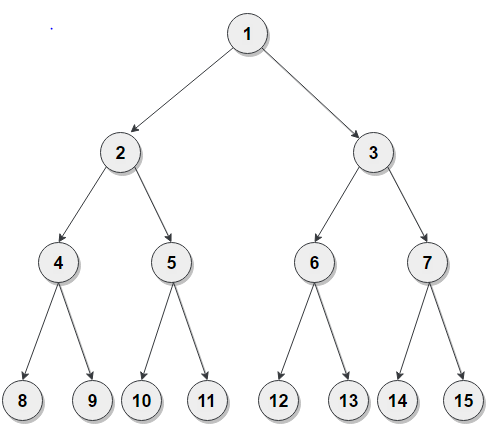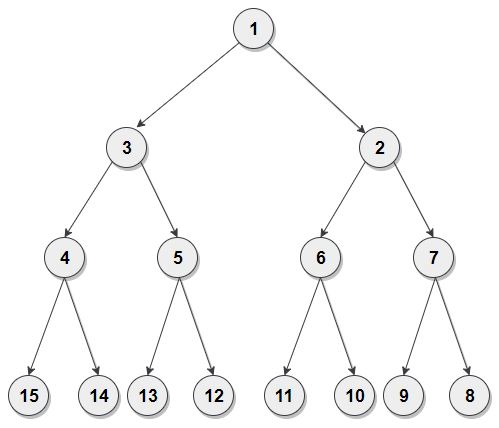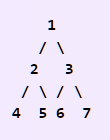Problem title
Difficulty
Avg time to solve

Search In A Grid
Moderate
15 mins
Word Pattern
Easy
15 mins
Make Palindrome
Easy
--
Gas Stations
Moderate
10 mins
Number of GP sequence
Moderate
10 mins
Perfect Number
Easy
10 mins
Form the Biggest Number
Moderate
25 mins
Check Square
Moderate
10 mins
Sorted Subsequence of Size 3
Moderate
15 mins
Remove maximum edges
Easy
15 mins2

# Reverse Alternate Nodes

Difficulty: MEDIUM
Avg. time to solve
36 min
Success Rate
51%

Problem Statement

#### A perfect binary tree is a binary tree in which all the interior nodes have two children, and all leaves have the same depth or same level.

##### Example :
``````Given binary tree :
````````````After the reversal of alternate nodes, the tree will be :
``````##### Input Format :
``````The first line contains elements of the given binary tree in the level order form. The line consists of values of nodes separated by a single space. In case a node is null, we take -1 in its place.

For example, the input for the tree depicted in the below image would be :
````````````1
2 3
4 5 6 7
-1 -1 -1 -1 -1 -1 -1 -1
``````

#### Explanation :

``````Level 1 :
The root node of the tree is 1

Level 2 :
Left child of 1 = 2
Right child of 1 = 3

Level 3 :
Left child of 2 = 4
Right child of 2 = 5
Left child of 3 = 6
Right child of 3 = 7

Level 4 :
Left child of 4 = null (-1)
Right child of 4 = null (-1)
Left child of 5 = null (-1)
Right child of 5 = null (-1)
Left child of 6 = null (-1)
Right child of 6 = null (-1)
Left child of 7 = null (-1)
Right child of 7 = null (-1)

The first not-null node(of the previous level) is treated as the parent of the first two nodes of the current level. The second not-null node (of the previous level) is treated as the parent node for the next two nodes of the current level and so on.
The input ends when all nodes at the last level are null(-1).
``````
##### Note :
``````The above format was just to provide clarity on how the input is formed for a given tree.
The sequence will be put together in a single line separated by a single space. Hence, for the above-depicted tree, the input will be given as :

1 2 3 4 5 6 7 -1 -1 -1 -1 -1 -1 -1 -1
``````
##### Output Format :
``````Print in a single line 'N' single space-separated integers denoting the inorder traversal of the modified tree.
``````
##### Note :
``````You do not need to print anything. It has already been taken care of, just implement the given function.
``````
##### Constraints :
``````0 <= N <= 5*10^5
0 <= node.data <= 10^7 and node.data != -1

Time Limit : 1 sec
``````
##### Sample Input 1 :
``````1 2 3 4 5 6 7 -1 -1 -1 -1 -1 -1 -1 -1
``````
##### Sample Output 1 :
``````4 3 5 1 6 2 7
``````
##### Explanation For Sample Input 1 :
``````We don’t reverse the first level, but we will reverse the second level. So the value of the second level becomes {3, 2}, and we also don’t reverse the third level.
``````
##### Sample Input 2 :
``````1 2 3 4 5 6 7 8 9 10 11 12 13 14 15 -1 -1 -1 -1 -1 -1 -1 -1 -1 -1 -1 -1 -1 -1 -1 -1
``````
##### Sample Output 2 :
``````15 4 14 3 13 5 12 1 11 6 10 2 9 7 8
``````
##### Explanation For Sample Input 2 :
``````We will reverse the second level, so values at the second level become {3, 2}, then we will reverse the fourth level, so values at the fourth level become {15, 14, 13, 12, 11, 10, 9, 8}.
``````Console